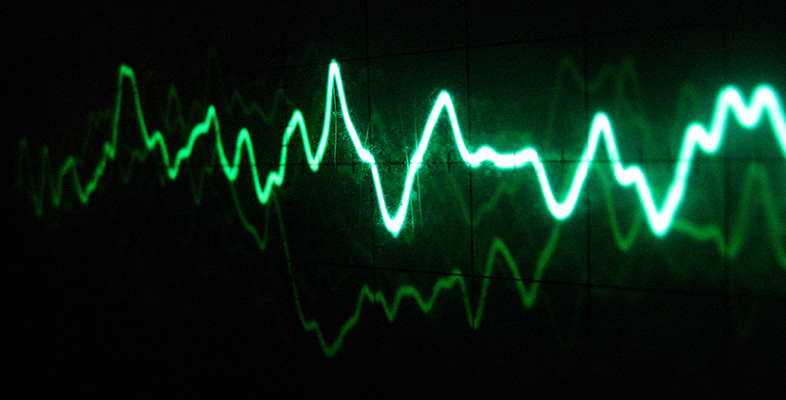Sound for music technology: An introduction

Start this free course now. Just create an account and sign in. Enrol and complete the course for a free statement of participation or digital badge if available.

Free course

# Sound for music technology: An introduction

A feature of decibels is that adding two decibel values is equivalent to multiplying the ratios they represent. To see how this comes about, consider another context in which a decibel measurement is often used, that of signal amplification.

In Figure 28, the triangular symbol represents an amplifier that amplifies a signal one-thousandfold. A sine wave enters the amplifier on the left and emerges on the right with its amplitude enlarged. (The sine waves are not drawn to scale.) The ratio of the output voltage amplitude to the input voltage amplitude is 1000:1; so, from Table 1, we can say that the voltage amplification is 60 dB. Such an amplifier is sometimes said to have a voltage gain of 1000, or 60 decibels.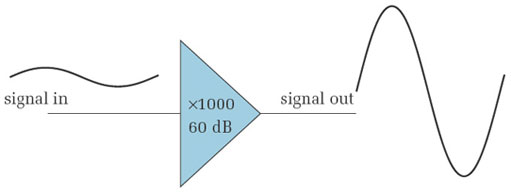Figure 28 A single-stage amplifier

An amplification of a thousand times could also be achieved in two separate stages, as shown in Figure 29. The first stage gives a tenfold amplification, and the second gives a one-hundredfold amplification. In terms of decibels, the first stage gives a gain of 20 dB and the second a gain of 40 dB. Adding these gives 60 dB, which we saw in Figure 28 (or Table 1) to be the decibel equivalent of an amplitude ratio of 1000:1. Thus adding decibels is equivalent to multiplying their corresponding ratios.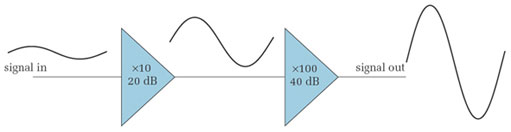Figure 29 A two-stage amplifier

## Activity 32 (Self-Assessment)

• (a) A two-stage amplifier consists of a stage that gives a gain of 12 decibels followed by a stage that gives a gain of 18 decibels. What is the overall amplification of the amplifier, both in decibels and as a ratio of natural numbers?

• (b) A two-stage amplifier has a first stage that amplifies by a factor of 8 and a second that amplifies by a factor of 10. What is the overall amplification of the amplifier, both in decibels and as a ratio of natural numbers?

• (a) The overall amplification is 12 dB + 18 dB = 30 dB. From Table 1 this can be seen to be equivalent to a ratio of 32:1. Multiplying the amplifications of the individual stages confirms this. The first gives an amplification ratio of 4:1 and the second an amplification ratio of 8:1.

• (b) The overall amplification ratio is 80:1. Table 1 does not give a decibel equivalent for this, but it must be the sum of the decibel equivalents for each stage. From Table 1 these are 18 dB and 20 dB, so the overall amplification is 18 dB + 20 dB, or 38 dB.

The property of decibels whereby adding them is equivalent to multiplying their corresponding ratios results from the fact that they are based on logarithms. Box 4 explains their mathematical background.

## Box 4: Mathematical definition of decibels

Decibels were originally devised as a way of expressing a ratio of powers. Given two powers P1 and P2, which have a ratio P1/P2, the decibel equivalent is defined to be: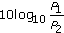For sound waves, the power is proportional to the square of the pressure amplitude. Hence, if the amplitudes corresponding to the power levels P1 and P2 are A1 and A2 respectively, the decibel equivalent of this power ratio can be written as: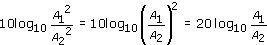This is the equation used to give the values in Table 2. If you have already worked with decibels as power ratios you might find the decibel values given in Table 1 are double what you are used to seeing for the corresponding ratios. The difference arises because Table 1 shows the decibel equivalents of amplitude ratios rather than power ratios.

TA212_1